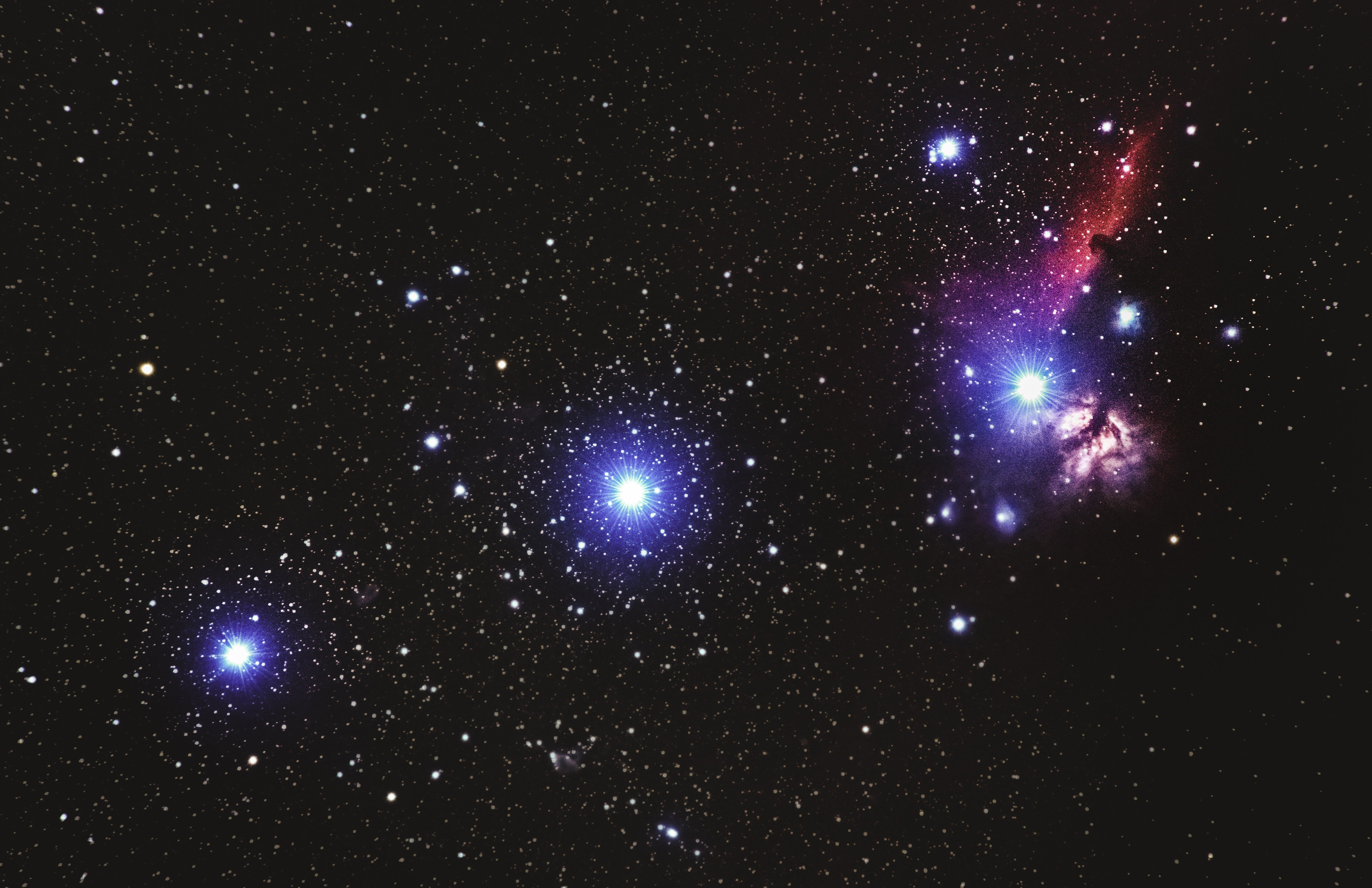Photo by Alexander Andrews on Unsplash

# The fault in the stars of Kotlin and Gson

`class A (p1:String,p2:String = "P2")`
`val a1 = A("x","y") // p1 = x, p2 = yval a2 = A("m") // p1 = m, p2 = P2`
`class A(@SerializedName("p1") val p1: String? = "P1",@SerializedName("p2") val p2: String? = "P2")class B(@SerializedName("p1") val p1: String? = "P1", @SerializedName("p2") val p2: String?)data class C(@SerializedName("p1") val p1: String? = "P1",@SerializedName("p2") val p2: String? = "P2")data class D(@SerializedName("p1") val p1: String? = "P1",@SerializedName("p2") val p2: String?)class E {@SerializedName("p1")val p1: String? = "P1"@SerializedName("p2")val p2: String? = "P2"}class F {@SerializedName("p1")var p1: String? = "P1"@SerializedName("p2")var p2: String? = "P2"}data class G(@SerializedName("p1")val p1: String? = "P1",@SerializedName("p2")val p2: String? = "P2")data class H(@SerializedName("p1")val p1: String?,@SerializedName("p2")val p2: String? = "P2")`
`val gson = Gson()print("\n")println("A : " + gson.toJson(gson.fromJson("{}",A::class.java)))print("\n")println("B : " + gson.toJson(gson.fromJson("{}",B::class.java)))print("\n")println("C : " + gson.toJson(gson.fromJson("{}",C::class.java)))print("\n")println("D : " + gson.toJson(gson.fromJson("{}",D::class.java)))print("\n")println("E : " + gson.toJson(gson.fromJson("{}",E::class.java)))print("\n")println("F : " + gson.toJson(gson.fromJson("{}",F::class.java)))print("\n")println("G : " + gson.toJson(gson.fromJson("{}",G::class.java)))print("\n")println("H : " + gson.toJson(gson.fromJson("{}",H::class.java)))print("\n")`
`A : {}B : {}C : {}D : {}E : {"p1":"P1","p2":"P2"}F : {"p1":"P1","p2":"P2"}G : {}H : {}`

## Solution:

Android Developer @ Cred

## More from AndroidPub

The (retired) Pub(lication) for Android & Tech, focused on Development